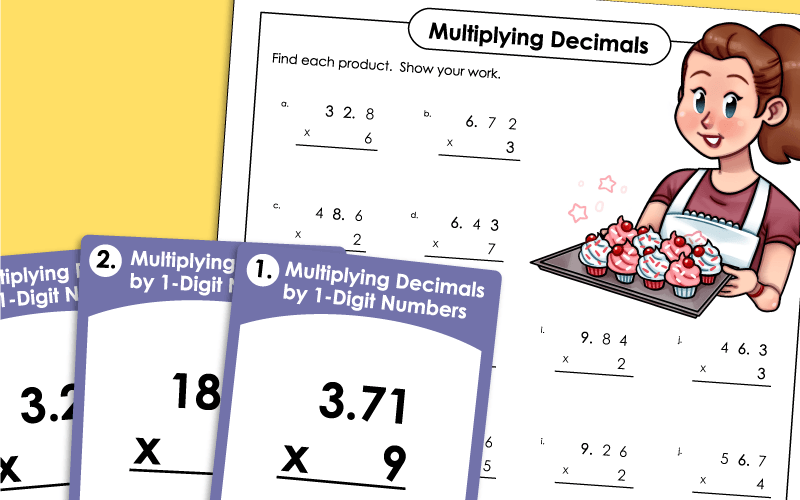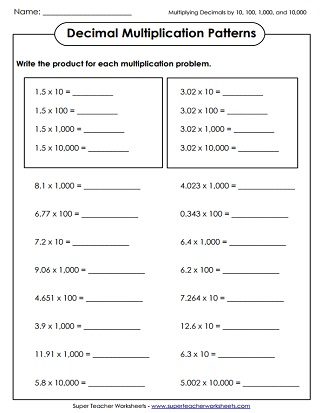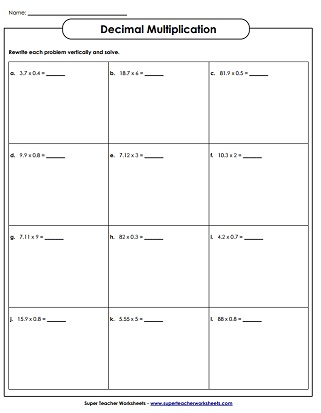# Multiplying Decimals

Use these worksheets to practice multiplying decimal factors together.## Multiplying DecimalsBy 1-Digit Numbers

This worksheet has 10 vertical problems and 2 word problems that students can solve to practice multiplying decimals by single digit numbers.
This worksheet has word problems to solve multiplying decimals by single digit numbers.
Solve each word problem by multiplying decimals to the tenths and hundredths place by single digit numbers.
Multiply each decimal by a one-digit number. (example: 18.7 x 6) This worksheet requires students to rewrite each problem vertically to solve.
Using this set of task cards, students will practice multiplying decimals to tenths and hundredths by single digit numbers.
Multiply each two digit number by decimals to the tenths and hundredths place.
Use these task cards to practice multiplying decimals by 10, 100 or 1,000.
The problems on this page were not solved correctly. Find the mistakes and explain how solve the problems correctly.

## Multiplying DecimalsBy 2-Digit Numbers

(example: 76.4 x 2.5)
Students will solve decimal by 2-digit number multiplication word problems on this worksheet.
Decide whether you need to multiply or divide to solve these decimal word problems.
Multiply decimals by 1 and 2-digit numbers. (examples: 3.4 x 4 and 1.35 x 5.5) Includes 8 vertical problems and 2 word problems.
Multiply the decimals inside the shapes to find the product.
These multiplication problems were not solved correctly. Find the mistakes, solve them correctly, and explain your thinking.
Multiply decimals by 2-Digit numbers. (example. 82 x 0.65) This worksheet requires students to rewrite problems vertically.
Students will use decimal multiplication to calculate their weight on all eight planets in the solar system.

## Decimal Multiplication Patterns

Multiply each decimal by 10; 100; 1000; 10,000; etc. (examples: 3.4x100, 5.671x10,000)
Find the products by multiplying decimals by 10, 100 and 1,000.
Students will find the missing factor or product for each decimal multiplication problem.

Dividing Decimals

Add and subtract pairs of decimal numbers.

## Sample Worksheet ImagesMy Account
Site Information